﻿ How to View the Mechanical Stress in Arbitrary DirectionExamples | Product | Murata Software Co., Ltd.Example52How to View the Mechanical Stress in Arbitrary Direction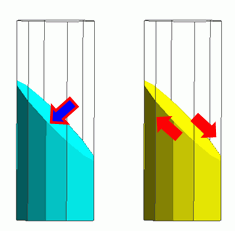General

• The mechanical stress is displayed in the direction specified in the Set Coordinate System.

• The deformation, the displacement and the mechanical stress are solved.

• Unless specified in the list below, the default conditions will be applied.

Analysis Space

 Item Settings Analysis Space 3D Model unit mm

Analysis Conditions

The default conditions are good enough for this exercise.

 Item Settings Solvers Mechanical Stress Analysis [Galileo] Analysis Type Static analysis Options N/A

Model

The model is a cylindrical solid body. The material is iron.
Distributed load is applied on the top face. The bottom face is fixedin Z direction.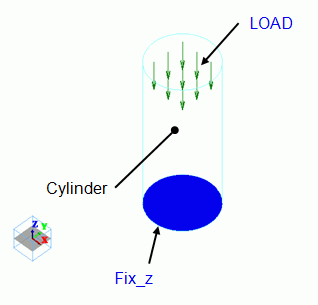Body Attributes and Materials

 Body Number/Type Body Attribute Name Material Name 0/Solid Cylinder 007_Fe *

* Available from the Material DB

Boundary Conditions

 Boundary Condition Name/Topology Tab Boundary Condition Type Settings Fix_z/Face Mechanical Displacement Select the Z component. UZ=0 LOAD/Face Mechanical Distributed face load X=0, Y=0, Z=-1.0×10^6[Pa]

Results

The contour indicates the Z normal stress. The unit is [Pa].

The maximum and minimum values for contour are set to 1 and -1 [MPa] respectively in the Graphics Setup dialog box.

To define the Section, enter (0, 0, 2.5) for Point on Cutting Plane and (0, 0, -1) for Normal Vector of Cutting Plane in the Section dialog box.

Also, select “Display the section and the surface simultaneously”.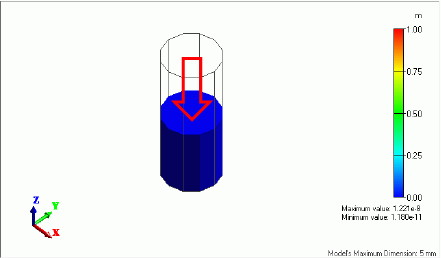Under this setting, the normal vector matches the direction of the stress component. Therefore, the normal compressive stress is shown.

If the section is slanted by 45° (Point on Cutting Plane: X = 0, Y = 0, Z = 2.5、Normal Vector: X = 1, Y = 0, Z = 1), you need to set the coordinate system to see the normal stress on the cutting plane or the shear stress along the cutting plane.

Select [Local Coordinate System] for the Coordinate System Type, and set up as follows.
Origin: X = 0, Y = 0, Z = 2.5, X’ Direction Vector: X = 1, Y = 0, Z = -1

By selecting Z’ normal stress, the stress component can be shown which is normal to the section slanted by 45 degrees.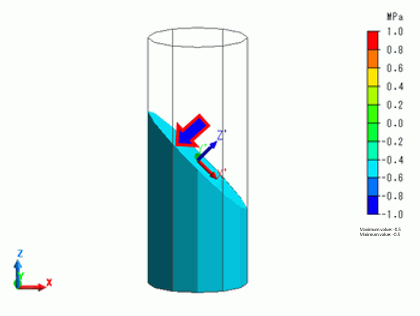The normal stress is -0.5[MPa].
The value is less than the applied load of -1MPa because it is in the slanted direction to the applied load direction.

By selecting Z’X’ shear stress, the shear stress can be shown along the section slanted by 45 degrees.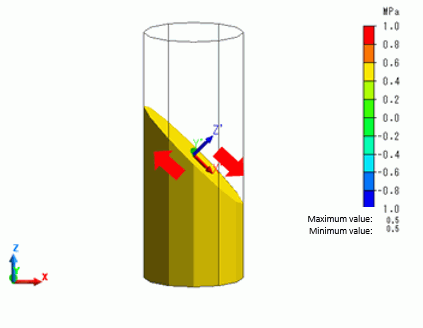The shear stress is 0.5[MPa].
In the diagram above, the red arrows indicate the force directions causing the shear deformation.

﻿